# # 第1节 数组理论基础

## # 二、数组的表现形式

### # 2.1 一维数组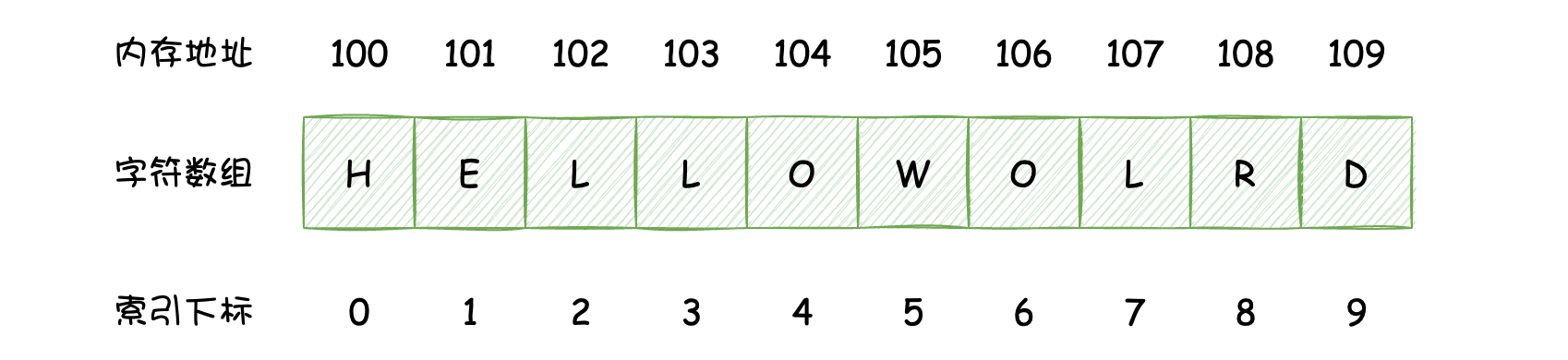• 这里的数组内存地址是虚拟描述的，是为了展现内存地址的连续性。
• 数组的索引下标都是从0开始的。

### # 2.2 多维数组

2.1中介绍的数组只有一个维度，它的每个元素都是最基本的数据，它被称为一维数组。如果一个数组中的元素的类型还是一个数组的话，那么这样的数组表现出来的就是多维数组，这里以二维数组为例，如下图所示：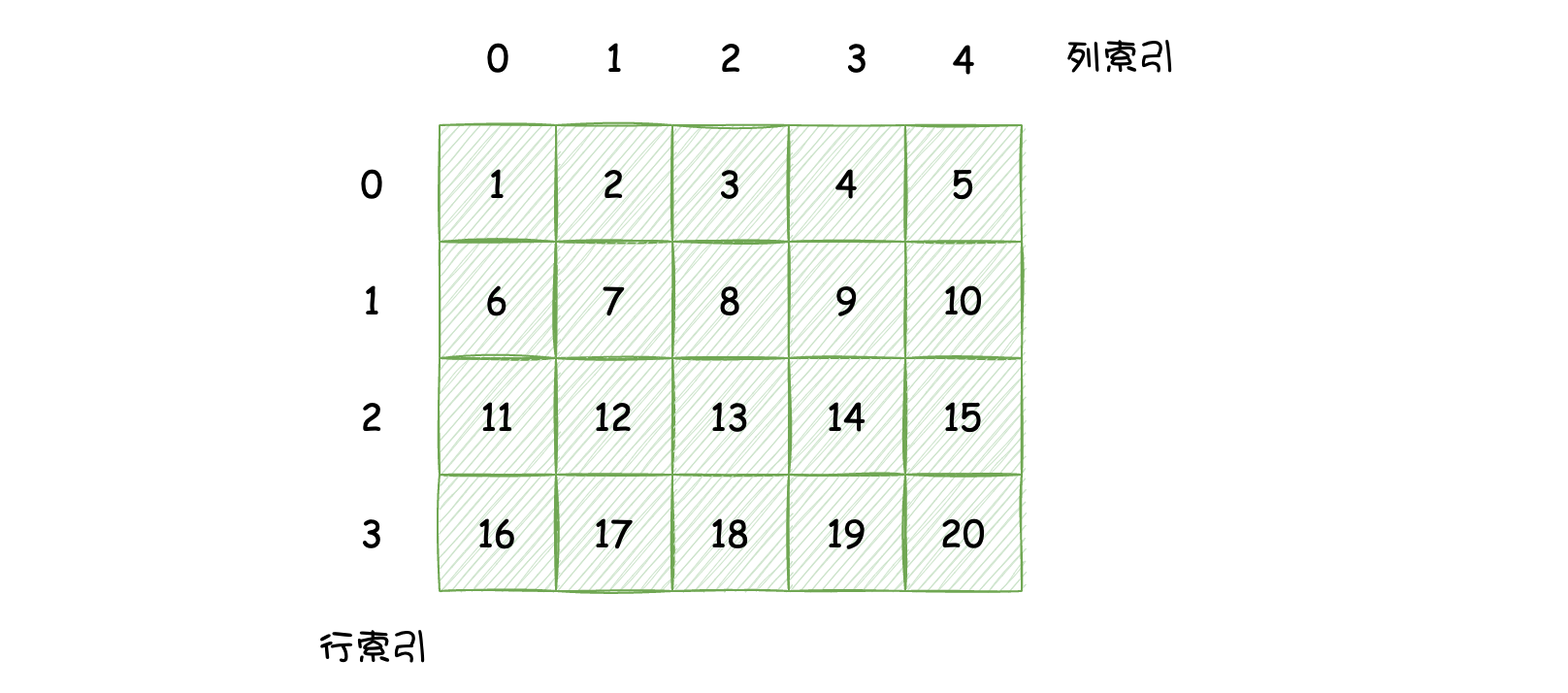### # 2.3 数组在Java中的实现

``````char[] helloWorld = new char[] {'H', 'E', 'L', 'L', 'O', 'W', 'O', 'R', 'L', 'D'};
``````

``````int[][] array = new int[][] {
{1, 2, 3, 4, 5},
{6, 7, 8, 9, 10},
{11, 12, 13, 14, 15},
{16, 17, 18, 19, 20}
};
``````

## # 三、数组的基本操作

### # 3.1 增：新增元素

``````public static void main(String[] args) {
char[] helloWorld = new char;
helloWorld = 'H';
helloWorld = 'E';
helloWorld = 'L';
helloWorld = 'L';
helloWorld = 'O';
helloWorld = 'W';
helloWorld = 'O';
helloWorld = 'R';
helloWorld = 'L';
helloWorld = 'D';
}
``````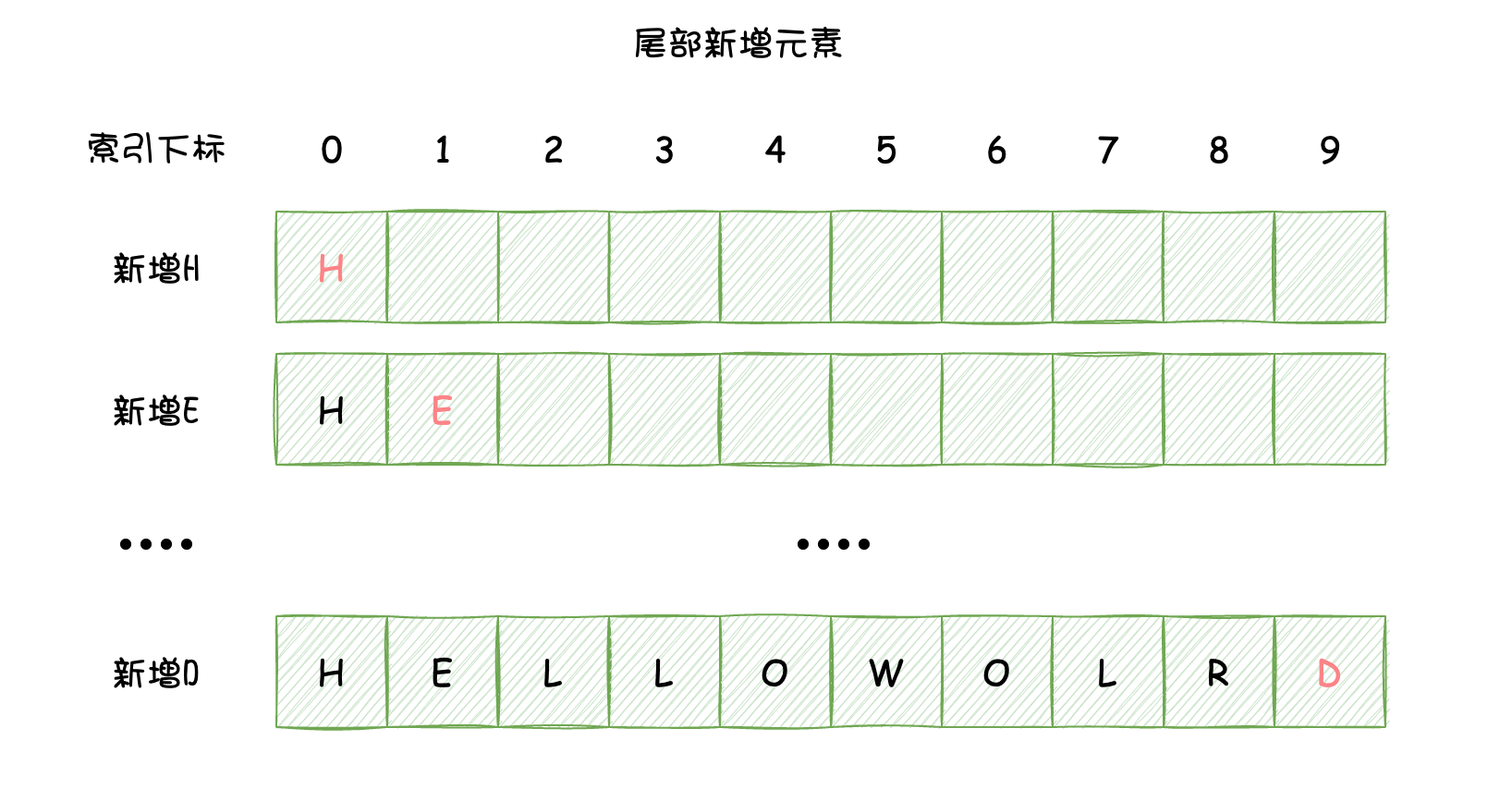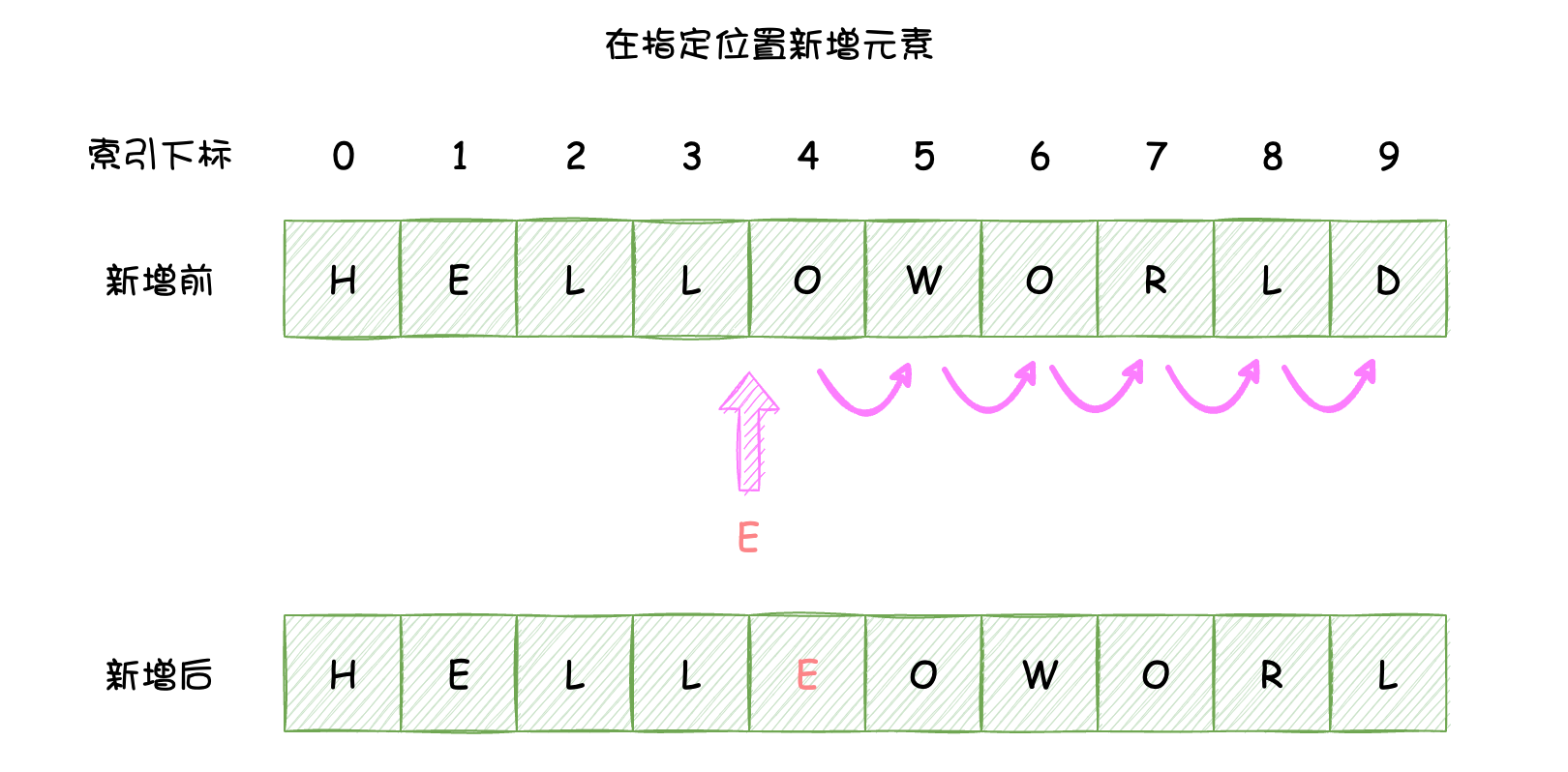``````public static void main(String[] args) {
char[] helloWorld = new char[] {'H', 'E', 'L', 'L', 'O', 'W', 'O', 'R', 'L', 'D'};
for (int i = helloWorld.length - 2; i >= 4; i--) {
helloWorld[i + 1] = helloWorld[i];
if (i == 4) {
helloWorld = 'E';
}
}
}
``````

### # 3.2 删：删除元素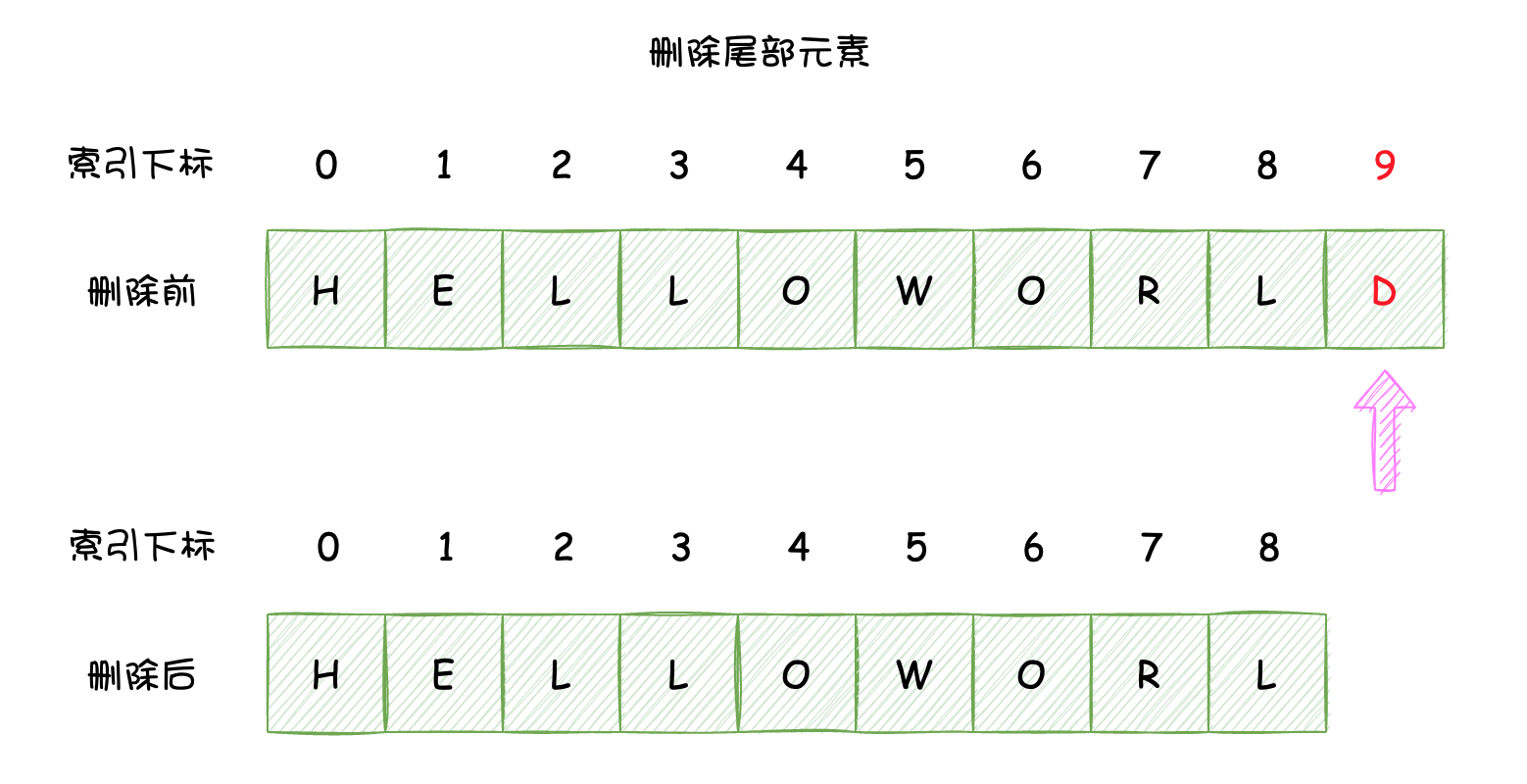``````public static void main(String[] args) {
char[] helloWorld = new char[] {'H', 'E', 'L', 'L', 'O', 'W', 'O', 'R', 'L', 'D'};
helloWorld = Arrays.copyOf(helloWorld, helloWorld.length - 1);
System.out.println(helloWorld);
}
``````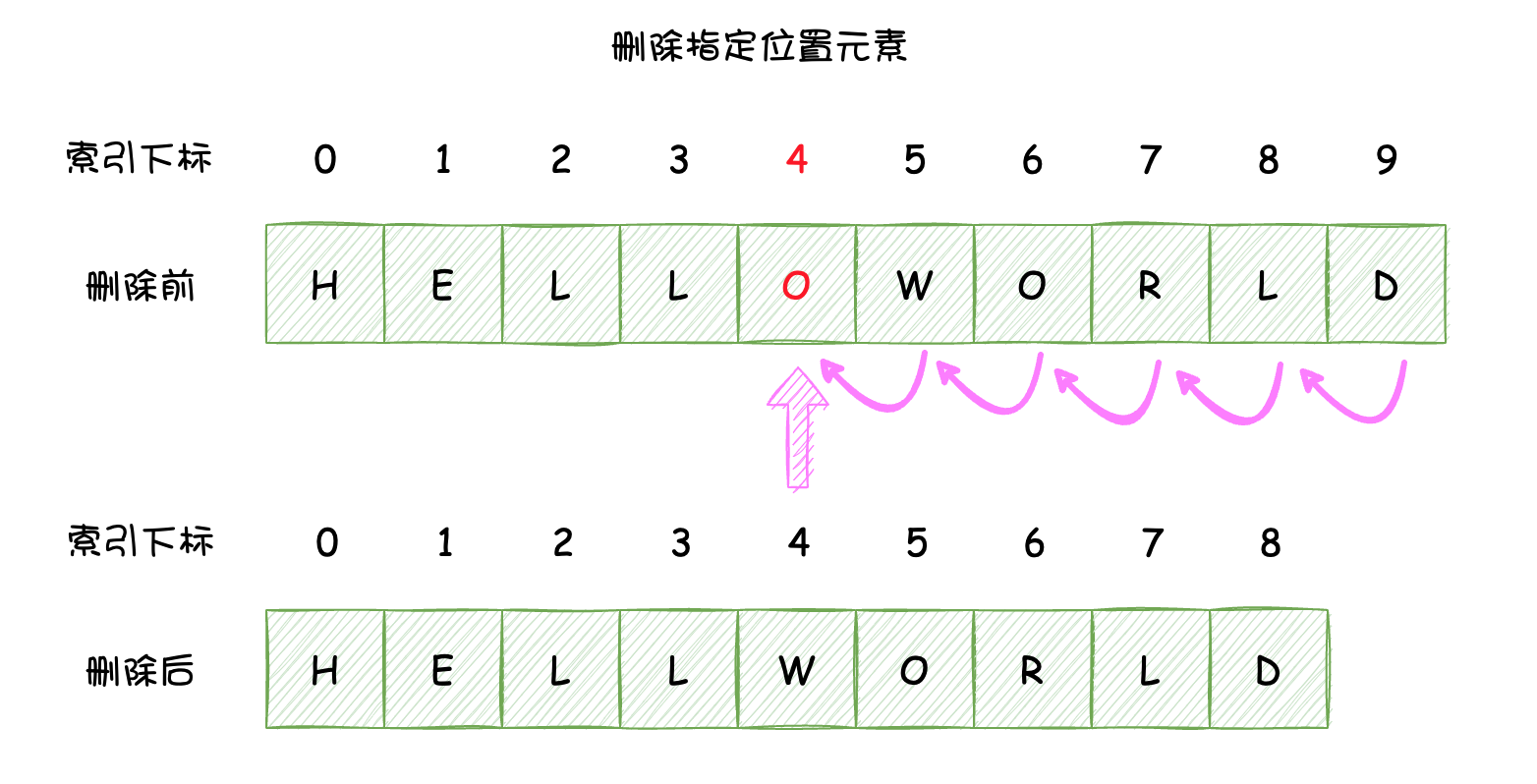``````public static void main(String[] args) {
char[] helloWorld = new char[] {'H', 'E', 'L', 'L', 'O', 'W', 'O', 'R', 'L', 'D'};
// 这里假设删除所以为4的元素，也就是O
for (int i = 5; i < helloWorld.length; i++) {
helloWorld[i -1] = helloWorld[i];
}
helloWorld = Arrays.copyOf(helloWorld, helloWorld.length - 1);
System.out.println(helloWorld);
}
``````

### # 3.3 改：修改元素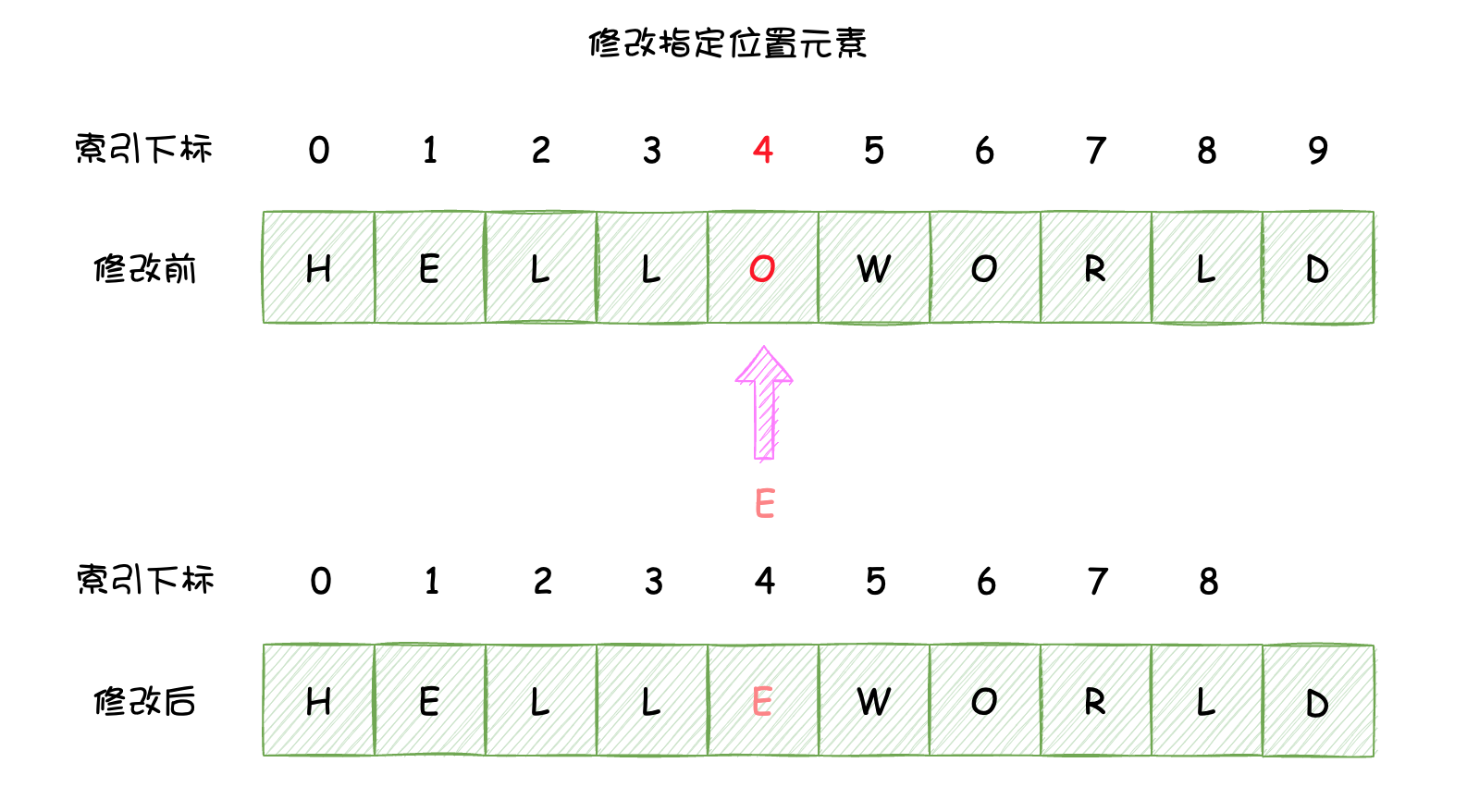``````public static void main(String[] args) {
char[] helloWorld = new char[] {'H', 'E', 'L', 'L', 'O', 'W', 'O', 'R', 'L', 'D'};
helloWorld = 'E';
System.out.println(helloWorld);
}
``````

### # 3.4 查：访问元素

``````public static void main(String[] args) {
char[] helloWorld = new char[] {'H', 'E', 'L', 'L', 'O', 'W', 'O', 'R', 'L', 'D'};
// 直接访问已知的位置的元素，时间复杂度：O(1)
System.out.println(helloWorld);
// 访问未知位置的第一个'O'元素，时间复杂度：O(n)
for (int i = 0; i < helloWorld.length; i++) {
if ('O' == helloWorld[i]) {
System.out.println('O');
break;
}
}
}
``````

## # 四、总结

• 数组的最大特点的支持随机访问；
• 数组访问元素、改变元素的时间复杂度为`O(1)`，在尾部插入、删除元素的时间复杂度也是`O(1)`，普通情况下插入、删除元素的时间复杂度为`O(n)`